# Loday algebra

(diff) ← Older revision | Latest revision (diff) | Newer revision → (diff)

Leibniz algebra

Loday algebras were introduced under the name "Leibniz algebras" by J.-L. Loday [a10] [a11] as non-commutative analogues of Lie algebras (cf. also Lie algebra). They are defined by a bilinear bracket which is no longer skew-symmetric. See [a12] for motivations, an overview and additional references. The term "Leibniz algebra" was used in all articles prior to 1996, and in many posterior ones. It had been chosen because, in the generalization of Lie algebras to Loday algebras, it is the derivation property of the adjoint mappings, analogous to the Leibniz rule in elementary calculus, that is preserved, while the skew-symmetry of the bracket is not. However, it has been shown [a1], [a8] that in many instances it is necessary to consider both a bracket and an associative multiplication defined on the same space, and to impose a "Leibniz rule" relating both operations, stating that the adjoint mappings are derivations of the associative multiplication. For this reason, it is preferable to adopt the term "Loday algebra" rather than "Leibniz algebra" when referring to the derivation property of the bracket alone.

A left Loday algebra over a fieldis a vector space overwith a-bilinear mapping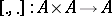satisfying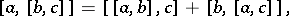for all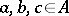. This property means that, for eachin, the adjoint endomorphism of,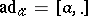, is a derivation of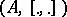.

Similarly, by definition, in a right Loday algebra, for each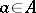, the mapping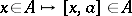is a derivation of.

A left or right Loday algebra in which the bracketis skew-symmetric (or alternating, ifis of characteristic) is a Lie algebra.

Loday algebra structures on a vector spacecan be defined as elements of squarewith respect to a graded Lie bracket on the vector space of-valued multi-linear forms on[a3].

A graded version of a left (or right) Loday algebra has been introduced by F. Akman [a1] and further studied in [a8]. The graded Loday algebras generalize the graded Lie algebras (cf. also Lie algebra, graded).

## Contents

### Examples.

The tensor module,, of any vector spacecan be turned into a Loday algebra such that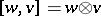, for,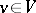. This is the free Loday algebra over.

Given any differential Lie algebra or, more generally, any differential left (respectively, right) Loday algebra,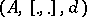, define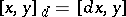(respectively,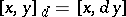). Thenis a left (respectively, right) Loday bracket, called the derived bracket [a8]. There is a generalization of this construction to the graded case, and the derived brackets on differential graded Lie algebras, which are graded Loday brackets, have applications in differential and Poisson geometry.

The operad associated to the notion of Loday algebra is a Koszul operad [a6]. There is a dual notion, the dual–Loday algebras, which are algebras over the dual operad.

### Loday (Leibniz) homology.

This is the homology of the complex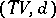with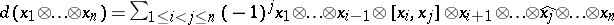, where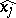denotes that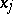is omitted. The homology complex of a Loday algebra is a co-algebra in the category of dual–Loday algebras.

The Loday homology of the algebra of matrices over an associative algebra, over a field of characteristic zero, is isomorphic to the tensor module of the Hochschild homology of(cf. also Extension of an associative algebra) as a group in the category of dual Loday algebras [a4] [a13] [a16]. This is the analogue of the Loday–Quillen–Tsygan theorem relating the Lie-algebra homology of matrices to the graded symmetric algebra over the cyclic homology of(cf. also Cyclic cohomology).

### Loday (Leibniz) cohomology.

The cohomology can be defined dually to the homology. The-cochains on a Loday algebra, with coefficients in a representationof(see [a10] [a13]), are the-linear mappings onwith values in, to which the differential of the Chevalley–Eilenberg complex can be lifted. Ifis the base fieldwith the trivial representation, the differential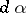of an-cochainis defined by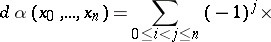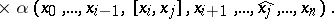### Di-algebras.

A di-algebra is an algebra with two associative operations satisfying additional axioms [a12]. A di-algebra is a non-commutative analogue of an associative algebra, and any di-algebra structure on a vector spacegives rise to a Loday-algebra structure on. The universal enveloping algebra of a Loday algebra [a13] has the structure of a di-algebra.

How to Cite This Entry:
Loday algebra. Encyclopedia of Mathematics. URL: http://encyclopediaofmath.org/index.php?title=Loday_algebra&oldid=18089
This article was adapted from an original article by Yvette Kosmann-Schwarzbach (originator), which appeared in Encyclopedia of Mathematics - ISBN 1402006098. See original article